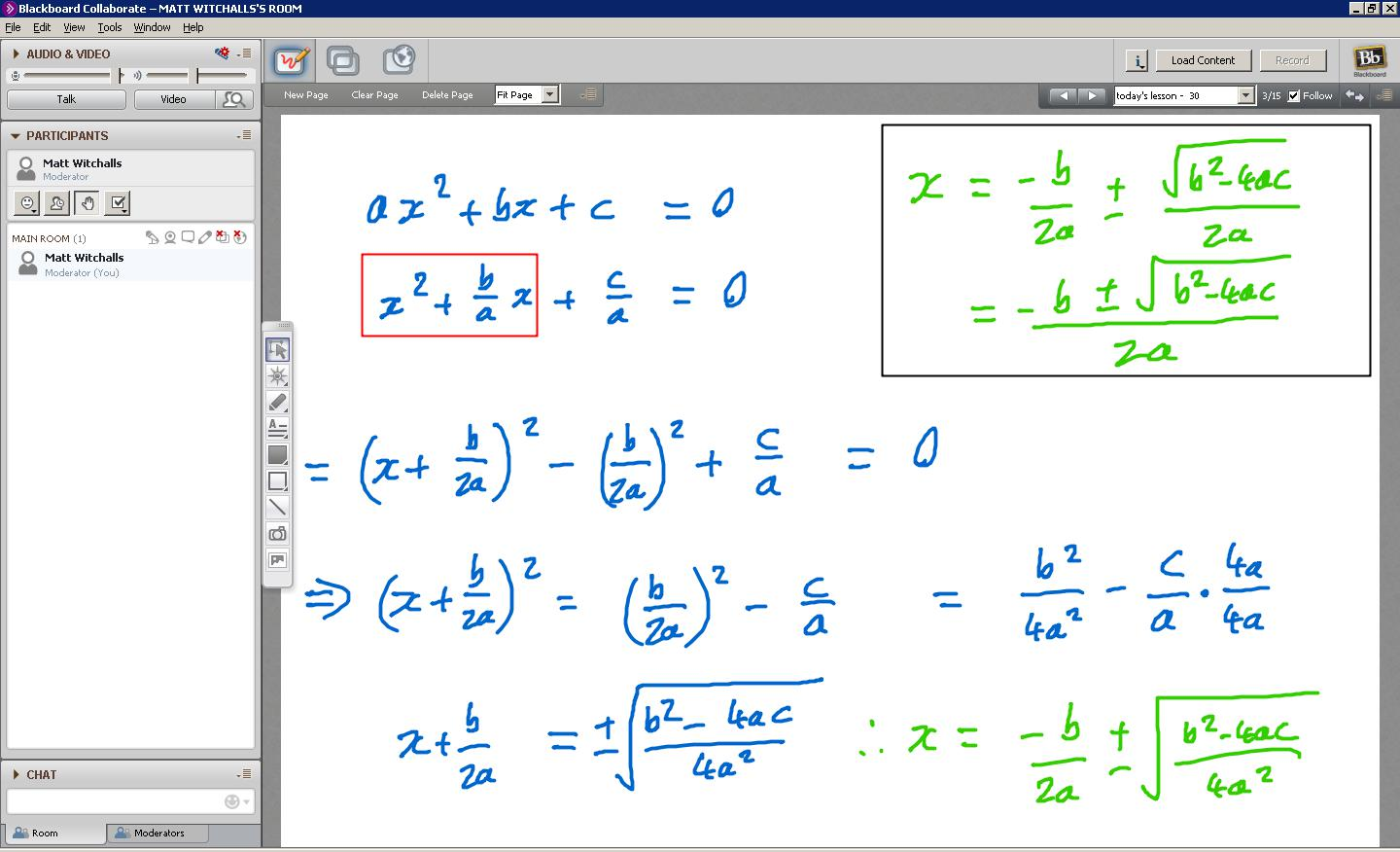## Ever wondered where the quadratic formula comes from?

Starting with the general form of a quadratic equation, you can derive the quadratic formula yourself using the technique of ‘completing the square’.As you can see in the above screenshot from a recent online maths lesson, it is possible to start with the general form of a quadratic equation$ax^2 + bx + c = 0$ and manipulate it into the classic quadratic formula$x = \frac{-b \pm \sqrt{b^2 - 4ac}}{2a}$.

The key to this derivation is the technique of completing the square. This is applied to the content of the red box in Line 2 and allows us to form the expression in Line 3.

Line 4 is all about making$(x + \frac{b}{2a})^2$ the subject and then creating an algebraic common denominator of$4a^2$ for the expression on the right-hand side.

Line 5 involves taking the square root of both sides and then$x$ is made the subject in the green equation in the bottom right corner.

Next, the box in the top right corner shows the square root being applied to the denominator of$4a^2$ to create a new common denominator of$2a$. Finally, the two algebraic fractions are combined and we’re done!$ax^2 + bx + c = 0 \rightarrow x = \frac{-b \pm \sqrt{b^2 - 4ac}}{2a}$

Would you like help with any of the maths in this post?